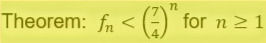top of page
Search
• vc9493

# Induction Proofs Applied to the Fibonacci Sequence

Updated: Mar 31

The Fibonacci sequence is defined using the recurrence formula:

fn+2 = fn+1 + fn for n ≧ 1 with f1 = 1 and f2 = 1.

The sequence begins 1, 1, 2, 3, 5, 8, 13, 21, 34, 55, 89, 144, 233, ...

Theorem: Every fourth number in the Fibonacci sequence is divisible by 3.

i.e. The subsequence f4n is divisible by 3 for n ≧ 1.

Proof: When n = 1, f4 = 3 which is divisible by 3.

Assume that the statement is true for n = k, so f4k is divisible by 3. Now,

3f4k+1 is divisible by 3 and so too is 2f4k using the inductive hypothesis. Hence f4(k+1) is divisible by 3 which means that the statement is true when n = k+1. So f4n is divisible by 3 for n ≧ 1 by mathematical induction.Now assume that the statement is true for n = i for all 1 ≤ i ≤ k for some k∈ℕ and k ≥ 2. In standard mathematical induction you have a single induction hypothesis; if you have multiple inductive hypotheses you can use “strong induction” to prove the result for n=k+1. So from our inductive hypotheses we can say that:

When n=k+1, we have

So the statement is true for n=k+1 and hence true for n ≥ 1 by strong induction.

Theorem: Binet's formula for the nth term of the Fibonacci sequence

Proof: First note that φ²＝φ+1, sinceSo two base cases are satisfied. Now assume that the statement is true for n = i for all 1 ≤ i ≤ k for some k∈ℕ and k ≥ 2. When n=k+1, we haveSo the statement is true for n=k+1 and hence true for n ≥ 1 by strong induction.

Theorem: Each term of the Fibonacci sequence can be expressed as a sum of binomial coefficients

Here the nCr terms are summed as long as n ≥ r.

Proof: This will require Pascal's combinatorial identity,

To see this,

Now, when n=1 and n=2, we have

So two base cases are satisfied. Now assume that the statement is true for n = i for all 1 ≤ i ≤ k for some k∈ℕ and k ≥ 2. When n=k+1, we have

So the statement is true for n=k+1 and hence true for n ≥ 1 by strong induction.